Apply a Gauss filter to an image with Python

• Last Updated : 26 Dec, 2020

A Gaussian Filter is a low pass filter used for reducing noise (high frequency components) and blurring regions of an image. The filter is implemented as an Odd sized Symmetric Kernel (DIP version of a Matrix) which is passed through each pixel of the Region of Interest to get the desired effect. The kernel is not hard towards drastic color changed (edges) due to it the pixels towards the center of the kernel having more weightage towards the final value then the periphery. A Gaussian Filter could be considered as an approximation of the Gaussian Function (mathematics). In this article we will learn methods of utilizing Gaussian Filter to reduce noise in images using Python programming language.

We would be using the following image for demonstration: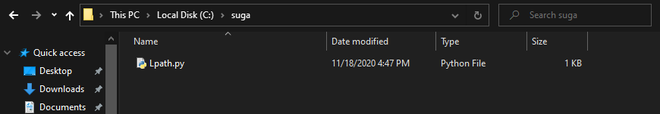A screenshot of a segment of windows explorer

Process to Apply a Gauss filter

In the process of using Gaussian Filter on an image we firstly define the size of the Kernel/Matrix that would be used for demising the image. The sizes are generally odd numbers, i.e. the overall results can be computed on the central pixel. Also the Kernels are symmetric & therefore have the same number of rows and column. The values inside the kernel are computed by the Gaussian function, which is as follows: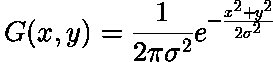2 Dimensional gaussian function

Where,

x → X coordinate value

y → Y coordinate value

????  → Mathematical Constant PI (value = 3.13)

σ → Standard Deviation

Using the above function a gaussian kernel of any size can be calculated, by providing it with appropriate values. A 3×3 Gaussian Kernel Approximation(two-dimensional) with Standard Deviation = 1, appears as follows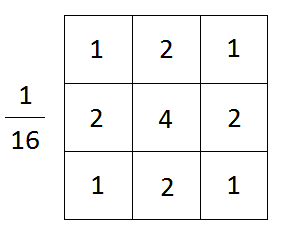Implementing the Gaussian kernel in Python

We would be using PIL (Python Imaging Library) function named filter() to pass our whole image through a predefined Gaussian kernel. The function help page is as follows:

Syntax: Filter(Kernel)

Takes in a kernel (predefined or custom) and each pixel of the image through it (Kernel Convolution).

Parameter: Filter Kernel

Return: Image Object

In the following example, we would be blurring the aforementioned image.

Python3

 # ImageFilter for using filter() functionfrom PIL import Image, ImageFilter  # Opening the image # (R prefixed to string in order to deal with '\' in paths)image = Image.open(r"IMAGE_PATH")  # Blurring image by sending the ImageFilter.# GaussianBlur predefined kernel argumentimage = image.filter(ImageFilter.GaussianBlur)  # Displaying the imageimage.show()

Output: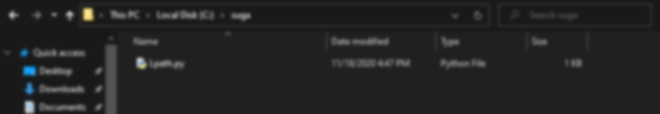Blurred Image

Explanation:

Firstly we imported the Image and ImageFilter (for using filter()) modules of the PIL library. Then we created an image object by opening the image at the path IMAGE_PATH (User defined). After which we filtered the image through the filter function, and providing ImageFilter.GaussianBlur (predefined in the ImageFilter module) as an argument to it. The kernel dimensions of ImageFilter.GaussianBlur is 5×5. In the end we displayed the image.

Note: The size of kernel could be manipulated by passing as parameter (optional) the radius of the kernel. This changes the following line from.

image = image.filter(ImageFilter.GaussianBlur)

to

where x => blur radius (size of kernel in one direction, from the center pixel)

Blurring a small region in an image:

Instead of the whole image, certain sections of it could also be selectively blurred. This could be performed by firstly cropping the desired region of the image, and then passing it through the filter() function. The output of which (the blurred sub image) would be pasted on top of the original image. This would give us the desired output.

The code for which is as follows:

Python3

 from PIL import Image, ImageFilter  image = Image.open(r"FILE_PATH")  # Cropping the image smol_image = image.crop((0, 0, 150, 150))  # Blurring on the cropped imageblurred_image = smol_image.filter(ImageFilter.GaussianBlur)  # Pasting the blurred image on the original imageimage.paste(blurred_image, (0,0))  # Displaying the imageimage.save('output.png')

Output: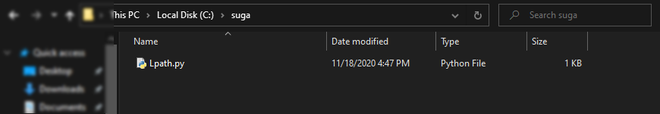Only the top left region of the image blurred

My Personal Notes arrow_drop_up